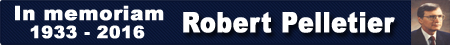# Conversion Factors

#### CSI Conversion Factors Explained

Long ago, when memory space was at a premium, price fields were represented as whole numbers which then had to be converted to a decimal or fractional representation. The conversion method was specific to the type of market. The native prices for some markets, such as grains, were in fractions. Others, like stock indices, were priced in decimals. CSI assigned a specific “conversion factor” to each market. These conversion factors are applied to the whole number values to produce decimal values.

##### For positive conversion factors, the conversion process is a straightforward shifting of the decimal place.
 0 = no modification +1 = division by 10 4321 = 432.1 +2 = division by 100 4321 = 43.21 +3 = division by 1,000 4321 = 4.321 +4 = division by 10,000 4321 = 0.4321 +5 = division by 100,000 4321 = 0.04321 +6 = division by 1,000,000 4321 = 0.004321

##### Negative conversion factors are used to convert to fractional values.
 -1 = right-most digit represents 8ths 4321 = 432 1/8 -2 = right-most two digits represent 16ths 4311 = 43 11/16 -3 = right-most two digits represent 32nds 10424 = 104 24/32 -4 = right-most two digits represent 64ths 10352 = 103 52/64 -5 = right-most three digits represent 128ths 104090 = 104 90/128 -6 = right-most three digits represent 256ths 104212 = 104 212/256 -7 = right-most three digits represent 32nds and halves of 32nds 103225 = 103 22.5/32 -8 = right-most three digits represent 32nds and eighths of 32nds 104222 = 104 22.25/32 -9 = right-most three digits represent 64ths and quarters 104222 = 104 22.25/64

##### Further explanation for –7, -8, -9 conversion factors:

These conversion factors were implemented to conform to the CBT method of displaying prices for certain instruments. For conversion factor –7, the final digit will always be 0 or 5. For conversion factor –8, the final digit will always be 0, 1, 2, 3, 5, 6, 7 or 8. For conversion factor -9, the final digit will always be 0, 2, 5, or 7. In each of these cases, the final digit represents the decimal value of the fractional portion, such that 0=.000, 1=.125, 2=.250, 3=.375, 5=.500, 6=.625, 7=.750 and 8=.875

##### Examples for conversion factors –7 and -8:

116060 means 116 and 6.000/32 (116.1875)
116061 means 116 and 6.125/32 (116.19140625)
116062 means 116 and 6.250/32 (116.1953125)
116063 means 116 and 6.375/32 (116.19921875)
116065 means 116 and 6.500/32 (116.203125)
116066 means 116 and 6.625/32 (116.20703125)
116067 means 116 and 6.750/32 (116.2109375)
116068 means 116 and 6.875/32 (116.21484375)

##### Examples for conversion factor –9:

116060 means 116 and 6.00/64 (116.09375)
116062 means 116 and 6.25/64 (116.09765625)
116065 means 116 and 6.50/64 (116.1015625)
116067 means 116 and 6.75/64 (116.10546875)